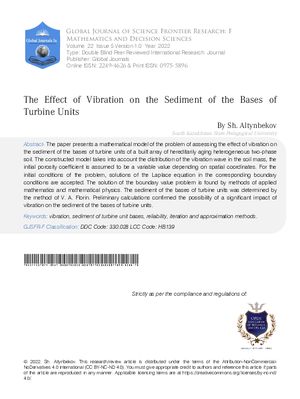### The Effect of Vibration on the Sediment of the Bases of Turbine Units

Authors

• Sh. Altynbekov

Keywords:

vibration, sediment of turbine unit bases, reliability, iteration and approximation methods

Abstract

The paper presents a mathematical model of the problem of assessing the effect of vibration on the sediment of the bases of turbine units of a built array of hereditarily aging heterogeneous two-phase soil The constructed model takes into account the distribution of the vibration wave in the soil mass the initial porosity coefficient is assumed to be a variable value depending on spatial coordinates For the initial conditions of the problem solutions of the Laplace equation in the corresponding boundary conditions are accepted The solution of the boundary value problem is found by methods of applied mathematics and mathematical physics The sediment of the bases of turbine units was determined by the method of V A Florin Preliminary calculations confirmed the possibility of a significant impact of vibration on the sediment of the bases of turbine units

How to Cite

Sh. Altynbekov. (2023). The Effect of Vibration on the Sediment of the Bases of Turbine Units. Global Journal of Science Frontier Research, 22(F5), 61–75. Retrieved from https://journalofscience.org/index.php/GJSFR/article/view/102578Published

2023-01-19

Issue

Section

Articles